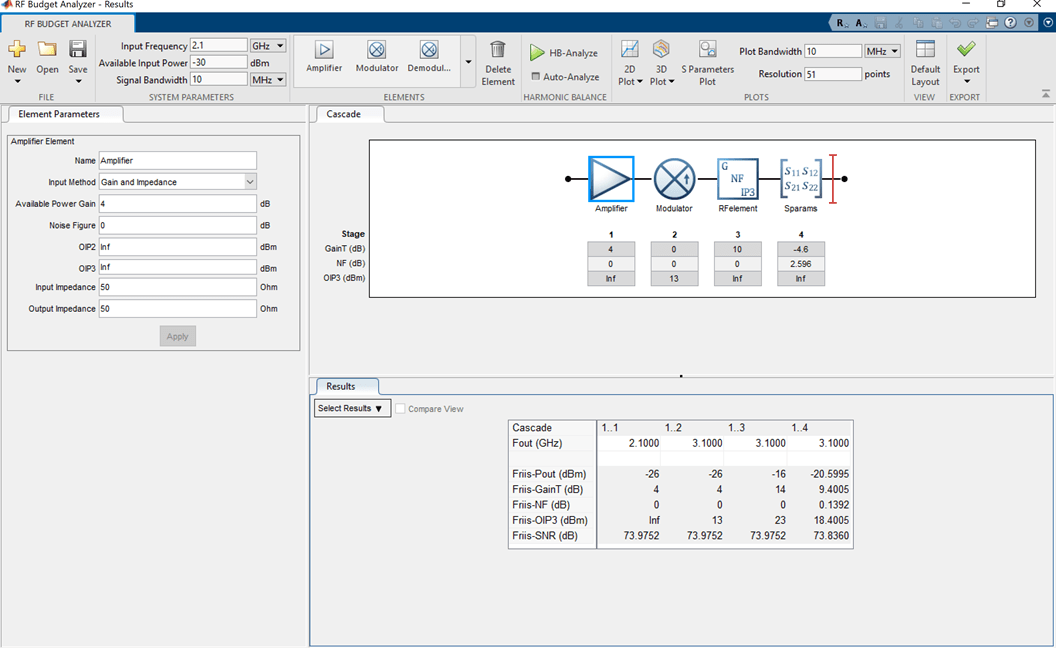# modulator

Modulator object

## Description

Use the `modulator` object to create a modulator element. A modulator is a 2-port RF circuit object. You can use this element in the `rfbudget` object and the `circuit` object.

## Creation

### Syntax

``mod = modulator``
``mod = modulator(Name=Value)``

### Description

example

````mod = modulator` creates a modulator object, `mod`, with default property values.```

example

````mod = modulator(Name=Value)` creates a modulator object with additional properties specified by one or more name-value pair arguments. Properties not specified retain their default values.```

## Properties

expand all

Name of modulator, specified as the comma-separated pair consisting of `'Name'` and a character vector. All names must be valid MATLAB® variable names.

Example: `Name='mod'`

Available power gain, specified as a nonnegative scalar in dB.

Example: `Gain=10`

Noise figure, specified as a real finite nonnegative scalar in dB.

Example: `NF=10`

Second -order output-referred intercept point, specified as a real scalar in dBm.

Example: `OIP2=8`

Third -order output-referred intercept point, specified as a real scalar in dBm.

Example: `OIP3=10`

Local oscillator frequency, specified as a real finite positive scalar in Hz.

Example: `LO=2e9`

Type of modulator, specified as `'Down'` or `'Up'`

Example: `ConverterType='Up'`

Ideal image reject filtering at the input of the modulator, specified as a numeric or logical `1 (true) or 0 (false)` . Setting this property to `false or 0` might affect harmonic balance results.

Example: `ImageReject=1`

Example: `ImageReject=true`

Ideal channel select filtering at the output of the modulator, specified as a numeric or logical `1 (true) or 0 (false)`. Setting this property to `false or 0` might affect harmonic balance results.

Example: `ChannelSelect=1`

Example: `ChannelSelect=false`

Input impedance, specified as a positive real part finite scalar in ohms. You can also use a complex value with a positive real part.

Example: `Zin=40`

Output impedance, specified as a scalar in ohms. You can also use a complex value with a positive real part.

Example: `Zout=40`

Number of ports, specified as a scalar integer. This property is read-only.

Names of port terminals, specified as a cell vector. This property is read-only.

## Object Functions

 `clone` Create copy of existing circuit element or circuit object

## Examples

collapse all

Create a downconverter modulator with a local oscillator (LO) frequency of 100 MHz.

`m = modulator(ConverterType='Down',LO=100e6)`
```m = modulator: Modulator element Name: 'Modulator' Gain: 0 NF: 0 OIP2: Inf OIP3: Inf Zin: 50 Zout: 50 LO: 100000000 ConverterType: 'Down' ImageReject: 1 ChannelSelect: 1 NumPorts: 2 Terminals: {'p1+' 'p2+' 'p1-' 'p2-'} ```

Create a modulator object with a gain of 4 dB and local oscillator (LO) frequency of 2 GHz. Create another modulator object that is an upconverter and has an output third-order intercept (OIP3) of 13 dBm.

```mod1 = modulator(Gain=4,LO=2e9); mod2 = modulator(OIP3=13,ConverterType='Up');```

Build a 2-port circuit using the modulators.

`c = circuit([mod1 mod2])`
```c = circuit: Circuit element ElementNames: {'Modulator' 'Modulator_1'} Elements: [1x2 modulator] Nodes: [0 1 2 3] Name: 'unnamed' NumPorts: 2 Terminals: {'p1+' 'p2+' 'p1-' 'p2-'} ```

Create an amplifier with a gain of 4 dB.

`a = amplifier(Gain=4);`

Create a modulator with an OIP3 of 13 dBm.

`m = modulator(OIP3=13);`

Create an N-port element using `passive.s2p`.

`n = nport('passive.s2p');`

Create an RF element with a gain of 10 dB.

`r = rfelement(Gain=10);`

Calculate the RF budget of a series of RF elements at an input frequency of 2.1 GHz, an available input power of –30 dBm, and a bandwidth of 10 MHz.

`b = rfbudget([a m r n],2.1e9,-30,10e6)`
```b = rfbudget with properties: Elements: [1x4 rf.internal.rfbudget.Element] InputFrequency: 2.1 GHz AvailableInputPower: -30 dBm SignalBandwidth: 10 MHz Solver: Friis AutoUpdate: true Analysis Results OutputFrequency: (GHz) [ 2.1 3.1 3.1 3.1] OutputPower: (dBm) [ -26 -26 -16 -20.6] TransducerGain: (dB) [ 4 4 14 9.4] NF: (dB) [ 0 0 0 0.1392] IIP2: (dBm) [] OIP2: (dBm) [] IIP3: (dBm) [ Inf 9 9 9] OIP3: (dBm) [ Inf 13 23 18.4] SNR: (dB) [73.98 73.98 73.98 73.84] ```

Type the `show` command at the command window to display the analysis in the RF Budget Analyzer app.

```show(b) ```## Version History

Introduced in R2017a